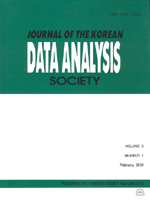상세검색
최근 검색어 전체 삭제
다국어입력
즐겨찾기0KCI등재 학술저널

# Connecting Partial Least Squares Regression and Generalized Ridge Regression

• 등재여부 : KCI등재
• 2005.02
• 61 - 71 (11 pages)

The main purpose of this paper is to make a connection between two regression methods which seemingly appear to be quite different: partial least squares regression (PLSR) and generalized ridge regression (GRR). The former uses latent factors extracted from both and and appears as an iterative algorithm in most literatures, and the latter adds small quantities to the diagonal of prior to inversion to reduce the variance of estimated regression coefficients. We connect the two methods to develop a new regression solution. This can be done by modifying PLSR expression in an approximate form and relating it to GRR expression, which results in a new type of estimates of ridge parameters. An example is given to illustrate the above method and its predictive ability is compared with other common regression methods.

1. Introduction

2. Expressions for GRR and PLSR Solutions

3. Connecting PLSR and GRR

4. An Example

5. Summary

References